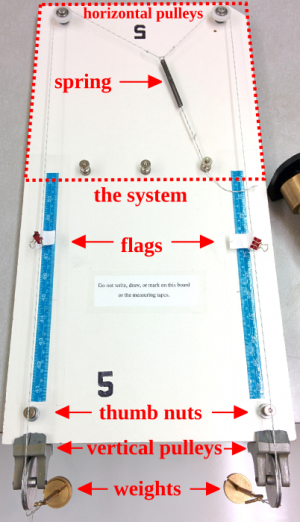### The Partial Derivative MachineWe have designed an apparatus, which we have called a Partial Derivative Machine (PDM), that serves as a mechanical analogue of a thermodynamic system. Using this device, students have a tangible way to wrestle with issues related to partial derivatives and thermodynamics, such as which variables are held fixed, how many variables are independent, and how energy can be added to a system.

The Partial Derivative Machine is an apparatus consisting of a spring system mounted on a large piece of particle board. This system can be manipulated by pulling on two strings, that extend to the front of the board, where they pass over pulleys and end at weight hangers. The tension in the strings may be manipulated by adding weights to the hangers, or alternatively their position may be held fixed by tightening the thumb nuts onto the string.

In order to more easily measure the state of the system, a measuring tape is placed, so that the numbers increase toward the user, on the board parallel to each string and flags are added to the strings. By numbering the two strings, the instructor is able to define four quantities for this experiment:

• $x_1$, the position of the flag on the first string
• $x_2$, the position of the flag on the second string
• $F_1$, the tension in the first string
• $F_2$, the tension in the second string

There are two conditions under which the system can be manipulated. The first method involves tightening one knob, thereby fixing $x_1$ or $x_2$, and then increasing the mass on the freely hanging string. For example, pinning the knob on the second string would fix $x_2$, then adding weight to the X string would increase $x_1$, $F_1$, and $F_2$.

Alternatively, one can leave both strings free and add weights to one or both. In doing so, placing weight on one string will (in general) cause the position of both strings to shift, changing both $x_1$ and $x_2$ as the system balances the forces. For example, adding weight to the first string would cause $x_1$ and $F_1$ to increase while $F_2$ stays constant and $x_2$ may either increase or decrease.

We note that it is often helpful to use a 'black box' to allow students to interact with the device first without seeing the internal mechanisms at work. This can prevent unproductive lines of work in which students attempt to predict the functional form of the variables rather than taking measurements.

For detailed information on building a PDM for yourself, including a parts list, see building a PDM

##### Views

New Users

Curriculum

Pedagogy

Institutional Change

Publications

##### Toolbox